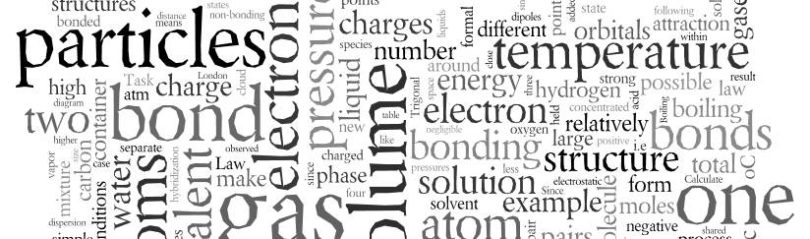# Hybridisation questions and answers### Hybridisation (Chemistry – Chemical Bonding) MCQs

Ques. Hybridization is due to the overlapping of
(a) Orbitals of different energy levels
(b) Orbitals of different energy content
(c) Orbitals of same energy content
(d) None of the above
Ans. (c)

Related: Trigonometry problems

Ques. The bond angle is minimum in
(a) H­2Te
(b) H2Se
(c) H2O
(d) H2S
Ans. (a)

Ques. The two types of bonds present in B2H6 are covalent and
(a) Three centre bond
(b) Hydrogen bond
(c) Two centre bond
(d) None of the above
Ans. (a)

Ques. The number of unpaired electrons in O2 molecule is
(a) 0
(b) 1
(c) 2
(d) 3
Ans. (c)

Related: Animal tissue class 11 mcq

Ques. In which compound, the hydrogen bonding is the strongest in its liquid phase
(a) HF
(b) HI
(c) CH4
(d) PH3
Ans. (a)

Ques. The structure of H2O2 is
(a) Planar
(b) Non-planar
(c) Spherical
(d) Linear
Ans. (b)

Ques. The two carbon atoms in acetylene are
(a) sp3 hybridized
(b) sp2 hybridized
(c) sp hybridized
(d) Unhybridized
Ans. (c)

Ques. Which one has sp2 –hybridization?
(a) CO2
(b) N2O
(c) SO2
(d) CO
Ans. (c)

Ques. Which species do not have sp3 hybridization?
(a) Ammonia
(b) Methane
(c) Water
(d) Carbon dioxide
Ans. (d)

Ques. The structure of PF5 molecule is
(a) Tetrahedral
(b) Trigonal bipyramidal
(c) Square planar
(d) Pentagonal bipyramidal
Ans. (b)

Ques. In diborane, the H – B – H bond angle is 120o. The hybridization of boron is likely to be
(a) sp
(b) sp2
(c) sp3
(d) dsp2
Ans. (c)

Ques. Shape of XeF­4 molecule is
(a) Linear
(b) Pyramidal
(c) Tetrahedral
(d) Square planar
Ans. (d)

Ques. The shape of IF7 molecule is
(a) Octahedral
(b) Pentagonal bipyramidal
(c) Trigonal bipyramidal
(d) Tetrahedral
Ans. (b)

Ques. The molecule of CO2 has 180° bond angle. It can be explanid on the basis of
(a) sp3 hybridisation
(b) sp2 hybridisation
(c) sp hybridization
(d) d2sp3 hybridisation
Ans. (d)

Ques. Compound formed by sp3d hybridization will have structure
(a) Planar
(b) Pyramidal
(c) Angular
(d) Trigonal bipyramidal
Ans. (d)

Related: Mechanical properties of fluids

Ques. Among the following compounds which is planar in shape
(a) Methane
(b) Acetylene
(c) Benzene
(d) Isobutene
Ans. (c)

Ques. Hybridisation involves
(a) Addition of an electron pair
(b) Mixing up of atomic orbitals
(c) Removal of an electron pair
(d) Separation of orbitals
Ans. (b)

Ques. XeF2 involves hybridization
(a) sp3
(b) sp3d
(c) sp3d2
(d) None of these
Ans. (b)

Ques. Compound having planar symmetry is
(a) H2SO4
(b) H2O
(c) HNO3
(d) CCL4
Ans. (c)

Ques. Number of shared electrons in between carbon-carbon atoms in ethylene molecule is
(a) 2
(b) 4
(c) 6
(d) 3
Ans. (b)

Ques. Which of the following molecules has trigonal planer geometry?
(a) IF3
(b) PCl3
(c) NH3
(d) BF3
Ans. (d)

Ques. The geometry and the type of hybrid orbital present about the central atom in BF3 is
(a) Linear, sp
(b) Trigonal planar, sp2
(c) Tetrahedral, sp3
(d) Pyramidal, sp3
Ans. (b)

Ques. Be in BeCl2 undergoes
(a) Diagonal hybridization
(b) Trigonal hybridization
(c) Tetrahedral hybridization
(d) No hybridization
Ans. (a)

Ques. The bond angle in water molecule is nearly or Directed bonds in water forms an angle of
(a) 120o
(b) 180o
(c) 109o 28’
(d) 104o 30’
Ans. (d)

Related: isomerism questions

Ques. When two pairs of electrons are shared, bond is?
(a) Single covalent bond
(b) Double covalent bond
(c) Dative bond
(d) Triple bond
Ans. (b)

Ques. sp3d2 hybrid orbitals are
(a) Linear bipyramidal
(b) Pentagonal
(c) Trigonal bipyramidal
(d) Octahedral
Ans. (d)

Ques. Carbon atoms in diamond are bonded to each other in a configuration
(a) Tetrahedral
(b) Planar
(c) Linear
(d) Octahedral
Ans. (a)

Ques. In benzene, all the six C – C bonds have the same length because of
(a) Tautomerism
(b) sp2 hybridization
(c) Isomerism
(d) Inductive effect
Ans. (b)

Ques. The nature of hybridization in the NH3 molecule is
(a) sp
(b) sp2
(c) sp3
(d) sp3d
Ans. (c)

Ques. The geometry of the molecule with sp3d2 hybridised central atom is
(a) Square planar
(b) Trigonal bipyramidal
(c) Octahedral
(d) Square pyramidal
Ans. (c)

### About the author

#### Richa Bhardwaj

Richa (B. Tech) has keen interest in Science and loves to teach students about it through lectures and assignments. She always try to use simple language and sentences while writing to make sure learner understands everything properly.

• Hammed says:

Thank you very much

• Rida Fatima says:

Good for practice

• Pragati Goyat says:

Nice practice questions

• Muhammad Afroz says:

Best